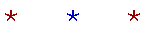An Approach

# CALCULUS

## The Lessons

The definition of a "continuous" quantity.
12.  Limits
A sequence of rational numbers.
The definition of the limit of a variable.
The limit of a function.
Theorems on limits. Limits of polynomials.
The definition of "a function is continuous at a value of x."
The definition of "a variable becomes infinite."
Limits of rational functions.
The slope of a tangent line to a curve.
The difference quotient and the definition of the derivative.
Notations for the derivative.
The equation of a tangent to a curve.
The derivative of a constant. The derivative of y = x.
The product rule.
The power rule.
The derivative of the square root function.
The derivative of a function of a function.
The quotient rule.
Implicit differentiation.
The derivative of inverse functions.
The second derivative.
The turning points of a graph. Critical values.
The system of natural logarithms.
The general power rule.#### Δημοσίευση σχολίου